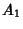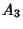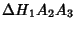Let,,, andbe four Points on a Circle, and,,,the Orthocenters of Triangles, etc. If, from the eight Points, four with different subscripts are chosen such that three are from one set and the fourth from the other, these Points form an Orthocentric System. There are eight such systems, which are analogous to the six sets of Orthocentric Systems obtained using the feet of the Angle Bisectors, Orthocenter, and Vertices of a generic Triangle.

On the other hand, if all the Points are chosen from one set, or two from each set, with all different subscripts, the four Points lie on a Circle. There are four pairs of such Circles, and eight Points lie by fours on eight equal Circles.

The Simson Line ofwith regard to Triangleis the same as that ofwith regard to the Triangle.